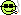1楼

2楼

3楼

4楼

(a-b)(b-c)(c-a)＝0。

＝a2(b-c)+b2(c-a)+c2(a-b)＋abc-abc
＝(a-b)(c-b)(c-a)＝0
∴a-b＝0，或c-b＝0，或c-a＝0，
∴a＝b，或b＝c，或c＝a，5楼

6楼

a . b .c 三个数至少有两个数相等的对立面即是这三个数都不相等，即a不等于b不等于c。

＝a^2(b-c)+b^2(c-a)-c^2[(b-c)+(c-a)]
＝(a^2-c^2)(b-c)+(b^2-c^2）(c-a)
＝(a-c)(a+c)(b-c)+(b-c)(b+c)(c-a)
＝(a-c)(b-c)[(a+c)-(b+c)]
＝(a-c)(b-c)(a-b)